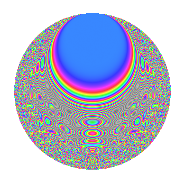Properties

 Label 4021.2.a.cLevel 4021 Weight 2 Character orbit 4021.a Self dual Yes Analytic conductor 32.108 Analytic rank 0 Dimension 182 CM No

Related objects

Newspace parameters

 Level: $$N$$ = $$4021$$ Weight: $$k$$ = $$2$$ Character orbit: $$[\chi]$$ = 4021.a (trivial)

Newform invariants

 Self dual: Yes Analytic conductor: $$32.1078466528$$ Analytic rank: $$0$$ Dimension: $$182$$ Fricke sign: $$-1$$ Sato-Tate group: $\mathrm{SU}(2)$

$q$-expansion

The dimension is sufficiently large that we do not compute an algebraic $$q$$-expansion, but we have computed the trace expansion.

 $$\operatorname{Tr}(f)(q) =$$ $$182q$$ $$\mathstrut +\mathstrut 18q^{2}$$ $$\mathstrut +\mathstrut 28q^{3}$$ $$\mathstrut +\mathstrut 208q^{4}$$ $$\mathstrut +\mathstrut 22q^{5}$$ $$\mathstrut +\mathstrut 18q^{6}$$ $$\mathstrut +\mathstrut 14q^{7}$$ $$\mathstrut +\mathstrut 54q^{8}$$ $$\mathstrut +\mathstrut 238q^{9}$$ $$\mathstrut +\mathstrut O(q^{10})$$ $$\operatorname{Tr}(f)(q) =$$ $$182q$$ $$\mathstrut +\mathstrut 18q^{2}$$ $$\mathstrut +\mathstrut 28q^{3}$$ $$\mathstrut +\mathstrut 208q^{4}$$ $$\mathstrut +\mathstrut 22q^{5}$$ $$\mathstrut +\mathstrut 18q^{6}$$ $$\mathstrut +\mathstrut 14q^{7}$$ $$\mathstrut +\mathstrut 54q^{8}$$ $$\mathstrut +\mathstrut 238q^{9}$$ $$\mathstrut +\mathstrut 7q^{10}$$ $$\mathstrut +\mathstrut 138q^{11}$$ $$\mathstrut +\mathstrut 47q^{12}$$ $$\mathstrut +\mathstrut 5q^{13}$$ $$\mathstrut +\mathstrut 72q^{14}$$ $$\mathstrut +\mathstrut 41q^{15}$$ $$\mathstrut +\mathstrut 256q^{16}$$ $$\mathstrut +\mathstrut 29q^{17}$$ $$\mathstrut +\mathstrut 35q^{18}$$ $$\mathstrut +\mathstrut 48q^{19}$$ $$\mathstrut +\mathstrut 56q^{20}$$ $$\mathstrut +\mathstrut 22q^{21}$$ $$\mathstrut +\mathstrut 19q^{22}$$ $$\mathstrut +\mathstrut 91q^{23}$$ $$\mathstrut +\mathstrut 46q^{24}$$ $$\mathstrut +\mathstrut 230q^{25}$$ $$\mathstrut +\mathstrut 88q^{26}$$ $$\mathstrut +\mathstrut 103q^{27}$$ $$\mathstrut +\mathstrut 15q^{28}$$ $$\mathstrut +\mathstrut 75q^{29}$$ $$\mathstrut +\mathstrut 18q^{30}$$ $$\mathstrut +\mathstrut 43q^{31}$$ $$\mathstrut +\mathstrut 116q^{32}$$ $$\mathstrut +\mathstrut 15q^{33}$$ $$\mathstrut +\mathstrut 13q^{34}$$ $$\mathstrut +\mathstrut 185q^{35}$$ $$\mathstrut +\mathstrut 364q^{36}$$ $$\mathstrut +\mathstrut 15q^{37}$$ $$\mathstrut +\mathstrut 53q^{38}$$ $$\mathstrut +\mathstrut 80q^{39}$$ $$\mathstrut -\mathstrut 13q^{40}$$ $$\mathstrut +\mathstrut 68q^{41}$$ $$\mathstrut +\mathstrut 32q^{42}$$ $$\mathstrut +\mathstrut 82q^{43}$$ $$\mathstrut +\mathstrut 259q^{44}$$ $$\mathstrut +\mathstrut 37q^{45}$$ $$\mathstrut +\mathstrut 13q^{46}$$ $$\mathstrut +\mathstrut 121q^{47}$$ $$\mathstrut +\mathstrut 53q^{48}$$ $$\mathstrut +\mathstrut 244q^{49}$$ $$\mathstrut +\mathstrut 93q^{50}$$ $$\mathstrut +\mathstrut 144q^{51}$$ $$\mathstrut -\mathstrut 16q^{52}$$ $$\mathstrut +\mathstrut 101q^{53}$$ $$\mathstrut +\mathstrut 47q^{54}$$ $$\mathstrut +\mathstrut 49q^{55}$$ $$\mathstrut +\mathstrut 199q^{56}$$ $$\mathstrut +\mathstrut 3q^{57}$$ $$\mathstrut +\mathstrut 4q^{58}$$ $$\mathstrut +\mathstrut 254q^{59}$$ $$\mathstrut +\mathstrut 24q^{60}$$ $$\mathstrut +\mathstrut 8q^{61}$$ $$\mathstrut +\mathstrut 37q^{62}$$ $$\mathstrut +\mathstrut 19q^{63}$$ $$\mathstrut +\mathstrut 326q^{64}$$ $$\mathstrut +\mathstrut 65q^{65}$$ $$\mathstrut +\mathstrut 41q^{66}$$ $$\mathstrut +\mathstrut 91q^{67}$$ $$\mathstrut +\mathstrut 50q^{68}$$ $$\mathstrut +\mathstrut 50q^{69}$$ $$\mathstrut +\mathstrut 5q^{70}$$ $$\mathstrut +\mathstrut 212q^{71}$$ $$\mathstrut +\mathstrut 77q^{72}$$ $$\mathstrut +\mathstrut 5q^{73}$$ $$\mathstrut +\mathstrut 101q^{74}$$ $$\mathstrut +\mathstrut 127q^{75}$$ $$\mathstrut +\mathstrut 22q^{76}$$ $$\mathstrut +\mathstrut 87q^{77}$$ $$\mathstrut -\mathstrut 20q^{78}$$ $$\mathstrut +\mathstrut 86q^{79}$$ $$\mathstrut +\mathstrut 71q^{80}$$ $$\mathstrut +\mathstrut 358q^{81}$$ $$\mathstrut -\mathstrut 20q^{82}$$ $$\mathstrut +\mathstrut 139q^{83}$$ $$\mathstrut -\mathstrut 30q^{84}$$ $$\mathstrut +\mathstrut 25q^{85}$$ $$\mathstrut +\mathstrut 82q^{86}$$ $$\mathstrut +\mathstrut 36q^{87}$$ $$\mathstrut -\mathstrut 8q^{88}$$ $$\mathstrut +\mathstrut 100q^{89}$$ $$\mathstrut -\mathstrut 87q^{90}$$ $$\mathstrut +\mathstrut 74q^{91}$$ $$\mathstrut +\mathstrut 171q^{92}$$ $$\mathstrut +\mathstrut 50q^{93}$$ $$\mathstrut -\mathstrut 13q^{94}$$ $$\mathstrut +\mathstrut 217q^{95}$$ $$\mathstrut +\mathstrut 42q^{96}$$ $$\mathstrut +\mathstrut 20q^{97}$$ $$\mathstrut +\mathstrut 47q^{98}$$ $$\mathstrut +\mathstrut 389q^{99}$$ $$\mathstrut +\mathstrut O(q^{100})$$

Embeddings

For each embedding $$\iota_m$$ of the coefficient field, the values $$\iota_m(a_n)$$ are shown below.

For more information on an embedded modular form you can click on its label.

Label $$a_{2}$$ $$a_{3}$$ $$a_{4}$$ $$a_{5}$$ $$a_{6}$$ $$a_{7}$$ $$a_{8}$$ $$a_{9}$$ $$a_{10}$$
1.1 −2.81003 −0.712978 5.89629 1.47211 2.00349 1.95505 −10.9487 −2.49166 −4.13669
1.2 −2.80453 3.34034 5.86536 −1.76340 −9.36806 −4.23059 −10.8405 8.15785 4.94549
1.3 −2.71768 −3.37653 5.38576 2.58064 9.17630 −1.70858 −9.20140 8.40093 −7.01334
1.4 −2.69235 −2.05539 5.24877 −1.71000 5.53383 −4.72268 −8.74684 1.22462 4.60392
1.5 −2.67927 −1.86893 5.17851 2.00029 5.00738 −3.62338 −8.51610 0.492900 −5.35934
1.6 −2.67278 1.10671 5.14375 3.24617 −2.95798 −3.43903 −8.40257 −1.77520 −8.67630
1.7 −2.60649 −2.80454 4.79381 −0.526210 7.31002 5.03978 −7.28206 4.86546 1.37156
1.8 −2.58741 0.104608 4.69469 −1.37313 −0.270664 −0.569668 −6.97226 −2.98906 3.55285
1.9 −2.58653 3.00156 4.69012 3.01819 −7.76362 1.43921 −6.95807 6.00937 −7.80662
1.10 −2.57672 −1.91417 4.63947 −2.65404 4.93228 −2.26992 −6.80118 0.664061 6.83870
1.11 −2.56968 2.97740 4.60327 3.17406 −7.65098 1.41939 −6.68959 5.86491 −8.15632
1.12 −2.53727 1.21389 4.43775 −4.40547 −3.07998 −1.31615 −6.18523 −1.52646 11.1779
1.13 −2.51665 0.669973 4.33353 4.29318 −1.68609 3.31221 −5.87268 −2.55114 −10.8044
1.14 −2.51316 2.70275 4.31595 0.484778 −6.79244 2.96790 −5.82035 4.30486 −1.21832
1.15 −2.46406 1.32162 4.07159 −2.41266 −3.25655 −2.12421 −5.10452 −1.25332 5.94493
1.16 −2.43939 2.42778 3.95064 −0.884079 −5.92232 −2.32249 −4.75837 2.89413 2.15662
1.17 −2.38633 −0.134750 3.69456 −0.899112 0.321557 −0.745765 −4.04378 −2.98184 2.14558
1.18 −2.37596 −0.498603 3.64520 −0.0295149 1.18466 0.657526 −3.90895 −2.75139 0.0701264
1.19 −2.35347 0.704714 3.53883 3.49583 −1.65853 1.05101 −3.62159 −2.50338 −8.22733
1.20 −2.33739 −2.27957 3.46338 1.15085 5.32823 1.11748 −3.42047 2.19642 −2.68998
See next 80 embeddings (of 182 total)
 $$n$$: e.g. 2-40 or 990-1000 Embeddings: e.g. 1-3 or 1.182 Significant digits: Format: Complex embeddings Normalized embeddings Satake parameters Satake angles

Inner twists

This newform does not have CM; other inner twists have not been computed.

Atkin-Lehner signs

$$p$$ Sign
$$4021$$ $$-1$$

Hecke kernels

This newform can be constructed as the kernel of the linear operator $$T_{2}^{182} - \cdots$$ acting on $$S_{2}^{\mathrm{new}}(\Gamma_0(4021))$$.